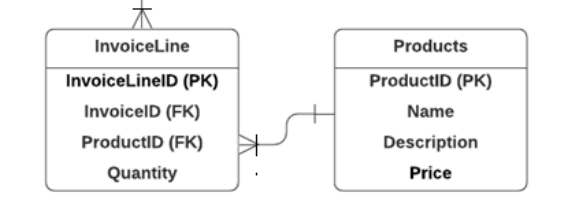# How to sum the values of a specific column attribute of individual rows returned using a LINQ expression? (C#)

I want to single out the rows of a table using a LINQ expression based off a certain passed value and then sum a specific column attribute of each of these rows while keeping the individual sums of the rows separate to one another.

The user enters a specific quantity value using a quantity input – this passed quantity value needs to be used in a LINQ expression to filter out the rows which meet the desired condition.

Below is a variable being assigned to the passed quantity value:

``````int quantitySelected;
JObject passedQuantity = JObject.Parse(data);
quantitySelected = (int)passedQuantity["qtySelect"]
``````

"quantitySelected" is now equal to the value passed through by the user – and now, using this value in a LINQ expression, i want to be able to filter a specific table (InvoiceLine) based off the condition being met and then include all the filtered rows according to a foreign key identifier (ProductID):

``````var dynamicReader = DBAccessor.InvoiceLines.Where(zz => zz.Quantity >= quantitySelected).Include(yy => yy.ProductID);
``````

The relevant extract from the database structure is as follows:I want to have all the rows which have a quantity greater or equal to what the user inputted, stored inside the dynamic reader with reference to the ProductID to which they are associated. So for example:I want to be able to single out the row which has a ProductID of 8 (which can be seen twice here) and then sum their quantities, (so ProductID 8 will ultimately have a sum of 6) and so forth (for each repeating ProductID).

Is there a way to accomplish this using a LINQ query expression? or will i need to use additional C#?